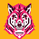# Moving Average Cross Alert, Multi-Timeframe (MTF) (by ChartArt)

46410 obejrzeń
See when two moving averages cross. With the option to choose between four moving average calculations:

The moving averages can be plotted from different time-frames, like e.g. the weekly or 4 hour time-frame using HL2 , HLC3 or OHLC4 as price source for the calculation. In addition there is a background color alert and arrows when the moving averages cross each other when the price also rises or falls. And the moving averages are colored depending on their trend direction (if they are trending up or down).
```study("Moving Average Cross Alert, Multi-Timeframe Option (MTF) (by ChartArt)", shorttitle="CA_-_MA_cross", overlay=true)

// ChartArt's Moving Average Cross Indicator
//
// Version 1.0
// Idea by ChartArt on September 15, 2015.
//
// This indicator shows when two moving averages cross.
// With the option to choose between four moving
// average calculations:
// (SMA = simple moving average)
// (EMA = exponential moving average)
// (WMA = weighted moving average)
// (Linear = Linear regression)
//
// The moving averages can be plotted from different
// timeframes, like the weekly or 4 hour timeframe.
// With the possibility to use HL2, HLC3 or OHLC4 prices.
//
// and arrows when the moving averages cross each other.
// And the moving averages are colored depending if
// they are trending up or down.
//
// List of my work:

// Multi-timeframe and price input
pricetype = input(close, title="Price Source For The Moving Averages")
useCurrentRes = input(true, title="Use Current Timeframe As Resolution?")
resCustom = input(title="Use Different Timeframe? Then Uncheck The Box Above", type=resolution, defval="W")
res = useCurrentRes ? period : resCustom
price = security(tickerid, res, pricetype)

// MA period input
shortperiod = input(50, title="Short Period Moving Average")
longperiod = input(100, title="Long Period Moving Average")

// MA calculation
smoothinput = input(2, minval=1, maxval=4, title='Moving Average Calculation: (1 = SMA), (2 = EMA), (3 = WMA), (4 = Linear)')
short = smoothinput == 1 ? sma(price, shortperiod) :
smoothinput == 2 ? ema(price, shortperiod) :
smoothinput == 3 ? wma(price, shortperiod) :
smoothinput == 4 ? linreg(price, shortperiod,0) :
na
long = smoothinput == 1 ? sma(price, longperiod) :
smoothinput == 2 ? ema(price, longperiod) :
smoothinput == 3 ? wma(price, longperiod) :
smoothinput == 4 ? linreg(price, longperiod,0) :
na

// MA trend direction color
shortcolor = short > short ? lime : short < short ? red : blue
longcolor = long > long ? lime : long < long ? red : blue

// MA output
MA1 = plot(short, title="Short Period Moving Average", style=linebr, linewidth=2, color=shortcolor)
MA2 = plot(long, title="Long Period Moving Average", style=linebr, linewidth=4, color=longcolor)
fill(MA1, MA2, color=silver, transp=50)

// MA trend bar color
TrendingUp() => short > long
TrendingDown() => short < long
barcolor(TrendingUp() ? green : TrendingDown() ? red : blue)

MAcrossing = cross(short, long) ? short : na
plot(MAcrossing, style = cross, linewidth = 4,color=black)

// MA cross background color alert
Uptrend() => TrendingUp() and TrendingDown()
Downtrend() => TrendingDown() and TrendingUp()
bgcolor(Uptrend() ? green : Downtrend() ? red : na,transp=50)

Buy = Uptrend() and close > close
Sell = Downtrend() and close < close
plotshape(Sell, color=black, style=shape.arrowdown, text="Sell", location=location.top)
```

## Komentarze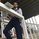Hi! Please can someone tell me how to add an alert for only when a sell or buy signal is generated, would be much appreciated! cheers.
Odpowiedz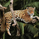Is it possible to have set this up as a 9 EMA and 20 SMA?
Odpowiedz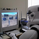I love this script and been using it for a little while but I was wondering how do I make an alert telling me when the buy and sell is?

Maybe I'm a bit confused with how to set it up in TradingView's alert system?
Odpowiedz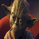Ddraig
@Ddraig, I would like to set up an alert when 4 time frames indicate a cross 2 with range candles 2 normal is this possible?
OdpowiedzDdraig
@Ddraig, The function creates alert condition that is available in Create Alert dialog. Please note, that alertcondition does NOT fire alerts from code automatically, it only gives you opportunity to create a custom condition for Create Alert dialog. Alerts must be still set manually. Also, an alert triggered based on a custom condition you created in Pine code is not displayed on a chart.
Odpowiedzmikeh21
@mikeh21, I had forgotten that I posted this, but you are correct and I have it working quite well for me.
Odpowiedz